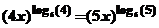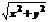WORCESTER POLYTECHNIC INSTITUTE

TWENTY-FIRST ANNUAL INVITATIONAL MATH MEET

OCTOBER 21, 2008

TEAM EXAM QUESTION SHEET

1.       Suppose  a  and  b  are digits (integers from 0 through 9).  What number divides  abba  for all choices of  a  and  b?

2.      Find all points  (x,y)  in the Euclidean plane satisfying  F(x,y) = 0  where

F(x,y) = xy4 – y4 + x3 y2 – x2 y2 – x3 + x2 – x +1

3.      Find  x > 0  satisfying the following equation4.      Arithmetic series sum:  200 + 205 + 210 + 215 + . . . 2745

5.      Factor as completely as possible over the reals the following polynomial:

x5 + x4 + 4 x3 – x2 – 19 x + 14

6.      A right circular cone is formed by cutting up a circular piece of paper which is 10 units in diameter.  A sector of angular measure  2Π/3  radians is removed and the remaining paper formed into the cone.  What is its volume?

7.      Hoodsie the cow is tethered to a corner of a barn which is 20′ by 40′.  Her rope is 60′ long.  How much grazing area does she have?  No barn doors are open.

8.      How many zeroes are at the end of the expansion of  31!

9.      A circle inscribed in an equilateral triangle and a square inscribed in the circle.  What is the ratio of the area of the triangle to that of the square?

10.   What is the minimum value ofif  5x + 12y = 60?

11.   The sum of an infinite geometric series with  -1< r < 1  as its common ratio, is 15.  The sum of the squares of the terms in this series is 45.  What is the first term in this series?

12.   How many permutations of the letters  D,O,R,E,M,I  do not contain the word DO, RE, MI; that is none of the words DO, RE and MI appears as consecutive letters?

13.   For some real number  r,  the polynomial  8x3 +4x2 - 42x - 45  is divisible by  (x – r)2.  What is  r ?

14.   How many real solutions are there to the equation:  x256 – 25632 = 0 ?